## Main

The capacitance of a classical conductor is determined solely by its geometry. When charged, the electrons distribute in space in a manner that minimizes their electrostatic energy. Quantum mechanics introduces extra energies that add new contributions to the capacitance. As the energies simply add and capacitance is inversely proportional to energy, these contributions add in series with the classical geometric capacitance (Cg) to yield the total capacitance, Ctot−1=Cg−1+Cdos−1+Cxc−1. The first contribution is caused by the kinetic energy of the electrons. Adding electrons to a conductor requires finite kinetic energy and therefore adds a term (Cdos) that reduces the total capacitance. The second contribution results from the correlated motion of electrons, which generally leads to reduction of their total electrostatic energy1,5,6. This adds a negative capacitance term (Cxc) that increases the total capacitance. The density dependence of these terms captures the fundamental properties of the quantum ground state and their measurements in two-dimensional systems7,8,9,10,11 established the role of interactions in the ground state.

In one dimension, the capacitance plays a special role as it also determines the properties of the excitations. Described within the Luttinger model12, the fundamental excitations are collective waves of spin or charge. Electronic interactions lift the degeneracy of these modes by enhancing the velocity of the charge excitations by a factor g−1, the inverse Luttinger parameter. This electrostatic effect is captured by the compressibility of the electronic gas13, or equivalently by its capacitance2,14. Thus, the central parameter of the Luttinger liquid theory is directly related to the capacitance by the simple relation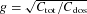. So far, g has been inferred mostly from transport measurements15,16,17,18,19,20 that probe the electronic excitations. Capacitance allows an independent thermodynamic determination of this parameter and its density dependence.

In this work we present the first direct capacitance measurements of carbon nanotubes (NT). A NT strongly coupled to a metallic gate forms a capacitor with a rigid geometry whose geometric and quantum capacitances are comparable, allowing us to extract both quantitatively. The measured geometrical capacitances are consistent with the classical expression for the capacitance between a gate and a wire. The observed quantum corrections demonstrate a symmetric one-dimensional sub-band structure of electrons and holes and yield g in the NT. Deviations of the measured capacitance from a theory that includes interactions only at Hartree mean-field level points to the importance of electron correlations.

We use top-gated semiconducting single-wall NT devices (Fig. 1a,b) in which the capacitor is formed between the top gate and the NT segment beneath it. The two side NT segments act as its leads. Figure 2 shows the measured transport of a top-gated device (T=77 K in all measurements reported in this paper). The source–drain conductivity, G=dI/dV, is plotted as a function of top-gate voltage, Vtg, which controls the density in the central NT segment, and back-gate voltage, Vbg, which tunes the density in the NT leads. The four corners in this plot are marked nnn, npn, pnp and ppp to reflect the carrier polarity in the NT leads and the central segment. Good conductivity is observed whenever the leads have the same polarity as the central segment (ppp and nnn corners). Therefore, to measure the capacitance in the n and p sides we tune the leads to be n or p type respectively to ensure that the contact resistance does not limit the charging of the NT (R100 GΩ).Figure 2: The measured transport across a top-gated semiconducting NT device.

Now we turn to the capacitance measurements and demonstrate how the NT capacitance is accurately differentiated from the background stray capacitance, C0. To this end, we exploit the tunability of the NT leads to carry out two independent capacitance measurements, one with and one without the NT central segment electrically connected to the measurement circuit. This is done by back-gating the NT leads into their semiconducting gap to increase their resistance to immeasurably large values. Consequently, the RC time to charge the central segment becomes too large and its capacitance drops from the measurement. In the inset of Fig. 3, we plot the capacitance measured as a function of Vbg at a fixed Vtg. The capacitance is constant at high Vbg, drops sharply around Vbg≈−4 V and remains constant at lower voltages. Comparing this with the transport measured on the same device (Fig. 2), the drop is associated with the leads becoming resistive (R100 GΩ). Thus, at low voltages the stray capacitance is measured. The NT capacitance, CNT, is then accurately determined from the measured capacitance step.

In Fig. 3 we show CNT measured in this manner for eight NT devices, plotted against the length of the NT extracted from the atomic force microscopy (AFM) images. We also plot the classical expression for the capacitance between a metallic wire and a metallic plane: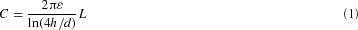where d and L are the wire diameter and length, h is the separation and the dielectric permittivity ɛ=3.9ɛ0 for SiOx. Two curves are shown, corresponding to d=1 nm and 4 nm, spanning the diameter range of our devices. We can see that the simple geometric formula describes the measured capacitances reasonably well.

Now we turn to the main result of this paper—the dependence of the NT capacitance on the carrier density, n. Figure 4a shows the measured capacitance as a function of Vtg. The cyan curve (NT leads pinched-off, Vbg=0) gives the baseline stray capacitance, which is independent of Vtg. In contrast, with n- or p-type leads (Vbg=+7 V/−7 V red/blue curves respectively) the capacitance has a well-pronounced structure—a large dip in the centre followed by symmetric structures at its positive and negative sides. Comparing this with the transport measured on the same device (Fig. 4b), the large dip is associated with the semiconducting gap. Outside the gap, the capacitance decreases slowly on both sides of the gap and then rises rapidly at symmetric locations with respect to the gap centre (Vtg≈+0.2 V,−3.6 V). At positive voltages we observe a second slow decrease following the sharp rise.Figure 4: NT capacitance and conductance as a function of the top-gate voltage.

Notably, there is a strong similarity between the voltage dependence of the capacitance on the electron and hole sides. To demonstrate this further, in Fig. 5 we overlay the measured capacitance in the electron side (red) with that of the hole side, reflected around the centre of the gap (blue). The two curves show an excellent agreement to within our measurement accuracy. This symmetry is notable if we realize that there is no inherent symmetry in the actual device parameters—at zero Vtg the device is strongly n type and therefore significantly different top-gate voltages are used to access the electron and hole sides.Figure 5: Detailed view of the top-gate dependence of NT capacitance and its comparison with theories.

The observed voltage dependence reflects the density of states of the one-dimensional sub-bands in the NT (Fig. 1d). Each sub-band gives rise to a sharp van-Hove singularity followed by a slow decrease of the density of states. These are manifested as sharp rises and slow decreases in the capacitance, where electron and hole sub-bands appear at opposite sides of the bandgap (qualitative evidence for sub-bands was recently seen21 in the local capacitance measured by AFM). The observed electron–hole symmetry of the capacitance reflects the underlying electron–hole symmetry in the band structure of the NT22 and demonstrates that it persists even in the presence of disorder.

To analyse our data quantitatively we begin with a simple Hartree model that consists of the density of states capacitance, a constant geometric capacitance and no electronic correlations: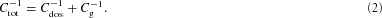The density of states capacitance at a given chemical potential, μ, and temperature, T, is given by: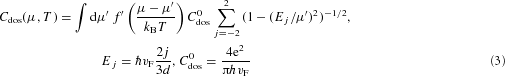where we included the first two electron and hole sub-bands, ħ is the reduced Planck’s constant, vF=8×105 m s−1 is the Fermi velocity and f′ is the derivative of the Fermi function. In the experiment, the total capacitance is measured as a function of gate voltage rather than the Fermi energy. We note that the conversion ratio between these two quantities is fully determined by the capacitances: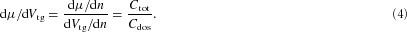Combining equations (2)–(4), we get an expression for the gate dependence of the total capacitance at finite temperatures, Ctot(Vtg,T), which is plotted for T=77 K in Figs 4a and 5 (dashed black line). By matching the theory and data at a single point (bottom of second sub-band step), we exhaust the free parameters of the theory, Cg and d, and the entire functional dependence is then fully determined. The overall structure of the measured capacitance is reproduced very well within the Hartree theory. The extracted parameters, d=2.35 nm and Cg=61.5 aF μm−1, compare favourably with the AFM-measured diameter d=2.5±0.5 nm and the capacitance C=76.5 aF μm−1 calculated with equation (1).

Near the semiconducting gap (−2.4 V<Vtg<−1 V) the measured capacitance deviates significantly from the theory. The reason becomes apparent when we compare it with the transport measured on the same device (Fig. 4b). The deviations are observed whenever the device is resistive (R≥100 GΩ) and consequently the capacitor does not charge completely. From comparison to theory, the capacitance roll-off happens at a finite density, n0≈200 e μm−1, corresponding to a Fermi energy of Ef30 mV. By comparing this with the transport data we conclude that the NT transistor turns off not when the tube is fully depleted of carriers, but when the barriers associated with disorder lead to an exponentially decreasing conductivity.

A fundamental quantity that can be readily determined from the capacitance measurement is g. In previous transport experiments15,16,17,18,19,20 this parameter has been deduced through its effects on the electronic excitations. Here we use the imprint of g on a thermodynamic property, the capacitance, to determine this parameter and its density dependence in a semiconducting NT. In the inset of Fig. 5 we plot the derivedas a function of the density of electrons (red) and holes (blue) in the first sub-band. The observed g changes continuously with density over a large range: g=0.26–0.34. These values are consistent with those deduced from transport experiments in NTs15,16,17,18 and predicted theoretically23,24.

Although the Hartree theory gives a reasonable account of the data, quantitative deviations can be seen when we compare them in detail (Fig. 5). In both sub-bands, the measured voltage dependence of the capacitance, dC/dVtg, is significantly steeper. In addition, the measured capacitance step at the opening of the second sub-band is significantly higher (40%). These deviations are much larger than our measurement uncertainty or any detectable deviations between electrons and holes. Adding disorder to the Hartree theory will decrease its predicted step height and will only increase the discrepancy between the theory and the measurement.

A possible explanation for the observed deviations is given by electronic exchange and correlations. These add a third term to the capacitance, Cxc, which is predicted2,3 to be negative at low densities. Consequently, whenever a new sub-band opens, it adds a new negative Cxc term to the capacitance. As this term adds in series, it will increase the total capacitance just after the opening of the sub-band, producing an upward step of the capacitance concurrent with the sub-band opening. This correlation-induced step should therefore increase the capacitance step beyond the Hartree prediction. This is exactly what we see in the opening of the second sub-band. It is also predicted2,3 that Cxc−1 decreases with increasing density and smoothly crosses over to positive values at high densities. This effect will decrease the total capacitance with density faster than expected from the Hartree theory, also in accordance with the data. To make a quantitative comparison, we add the leading correction to our theory by accounting for exchange effects (see Supplementary Information, note 1) and plot the resulting Hartree–Fock curve (green dashed line) in Fig. 5. Indeed, both the predicted C step and its derivative dC/dVtg become larger. However, although the Hartree–Fock approximation correctly captures the density dependence in the first sub-band, the predicted effect in the second sub-band is exaggerated compared with the measurement. Including the effect of disorder in the Hartree–Fock theory, which in contrast to the Hartree case will improve its agreement with the measurement, as well as a more complete treatment of electron correlations (Hartree–Fock is known to overestimate correlations) will be needed to explain our observations.

## Methods

### Device fabrication

The devices used in this study use a metallic top-gate separated from a NT by a thin layer of oxide to form a capacitor (Fig. 1a). The NTs were grown using chemical vapour deposition from patterned pads of evaporated Fe on degenerately doped silicon with a 200 nm SiO2 top layer. We used a C2H4/H2 growth recipe, which we found to produce predominantly single-walled NTs with small diameters (1–4 nm). After growth, the NTs were contacted with source and drain electrodes made of 50 nm of palladium, followed by aligned deposition of 10 nm SiOx dielectric and 50 nm aluminium top gate. This geometry enables us to carry out transport as well as capacitance measurements on the same device. All devices were imaged with AFM before their capacitance/transport measurements to ensure that only a single tube passes under the top gate. Figure 1b shows a typical image after the full fabrication sequence. All the measurements reported in this paper were done on semiconducting NTs at T=77 K.

### Capacitance measurements

The NT capacitances are measured using a capacitance bridge (Fig. 1c). The NT capacitor is embedded in the measurement arm of the bridge. A fixed a.c. voltage excitation (δ Vmeas=25–100 mV) along this arm is balanced against a second excitation along the reference arm (δ Vref) to null the voltage oscillations at the balance point B. Under these conditions, the measured capacitance is Cmeas=Crefδ Vref/δ Vmeas and the large stray capacitances between the leads of the NT capacitor and ground (CS1,CS2) are cancelled out. Direct capacitive coupling between the wiring is eliminated using shielded wires and by encapsulating the device in a Faraday cage. The direct capacitance between the on-chip gate and contact electrodes is shielded by a nearby back gate. After these steps, we are left with only a few hundred aF of fixed background capacitance. The measurements reported in this paper are done at 1 kHz. With a bridge sensitivity of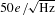and long averaging times we achieve a noise level smaller than 1 aF in these measurements.